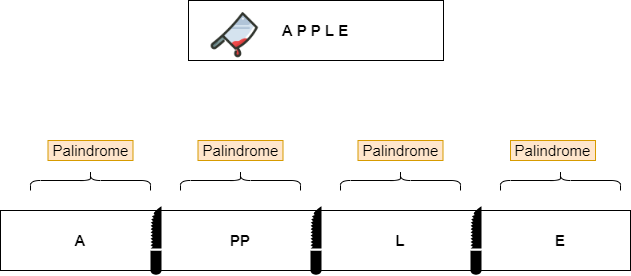New update is available. Click here to update.

# Palindrome Partitioning ll

Contributed by
Anup Kumar Singh
Last Updated: 23 Feb, 2023
Hard0/120
Avg time to solve 40 mins
Success Rate 60 %Share35 upvotes

## Problem Statement

Suggest Edit

#### The minimum number of cuts for the above example will be AA | CC | B. i.e 2 cuts

##### Note :
``````1) We can partition the string after the first index and before the last index.

2) Each substring after partition must be a palindrome.

3) For a string of length ‘n’, if the string is a palindrome, then a minimum 0 cuts are needed.

4) If the string contains all different characters, then ‘n-1’ cuts are needed.

5) The string consists of upper case English alphabets only with no spaces.
``````
Detailed explanation ( Input/output format, Notes, Images )##### Constraints :
``````1 <= T <= 50
1 <= length(string) <= 100

Where ‘T’ is the total number of test cases, ‘length(string)’ denotes the length of the string.

Time limit: 1 second
``````
##### Sample Input 1 :
``````3
NITIN
ABBAC
``````
##### Sample Output 1 :
``````4
0
1
``````
##### Explanation of sample input 1 :
``````Test case 1 :

For the first test case, we can do the cuts in the following way.

BAB | ABCBA | D | C  | EDE

After making the first cut at position 3, we get a substring ‘BAB’ which is a palindrome. Making the second cut at position 8, we get a substring ‘ABCBA’ which is a palindrome. Making the third cut at position 9, we get a char ‘D’, and furthermore making cuts at position 10 and 11 we get ‘C’ and ‘EDE’ respectively.

We can see after putting the partitions, every substring made by partitions is a palindrome and it is not possible to partition this string with less than 4 partitions such that each partition is a palindrome. Hence we return 4 which is the number of partitions to be made.

Test case 2 :

NITIN is already a palindrome so we do not need to do any cuts, Hence we return 0 which is the number of partitions to be made.

Test case 3 :

We can make cuts in the following way:

ABBAC  ->   ABBA | C

After making the first cut at position 4, we get the substring “ABBA” which is a palindrome. The remaining string is a single character ‘C’ which is a palindrome.

We can see after putting the partitions, every substring made by partitions are palindrome and it is not possible to partition this string with less than 1 partitions such that each partition is a palindrome
``````
##### Sample Input 2 :
``````3
CODINGNINJAS
APPLE
``````
##### Sample Output 2 :
``````7
2
3
``````
##### Explanation of sample input 2 :
``````Take the example of APPLE as shown below:

We can clearly see that we needed 3 cuts to partition the string into palindromic substrings.
``````AutoConsole